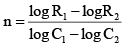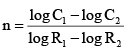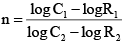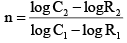Courses

# If the rate of a reaction are R1 and R2 at concentrations C1 and C2 of a reactant respectively. The order of reaction, ‘n’ (assuming that the concentrations of all other reactants and T remain constant) with respect to that reactant is given by:a)b)c)d)Correct answer is option 'B'. Can you explain this answer?

## Chemistry QuestionGaurav Singh Jul 26, 2018
As we know tha R=kc^n there fore R1=kc1^n and R2=kc2^n dividing both the equation we get R1/R2=(c1/c2)^n ..taking logarthimic of both the side we get log(R1/R2)=nlog(c1/c2) there fore n=logR1-logR2/logC1-logc2Girish Jha Nov 27, 2018